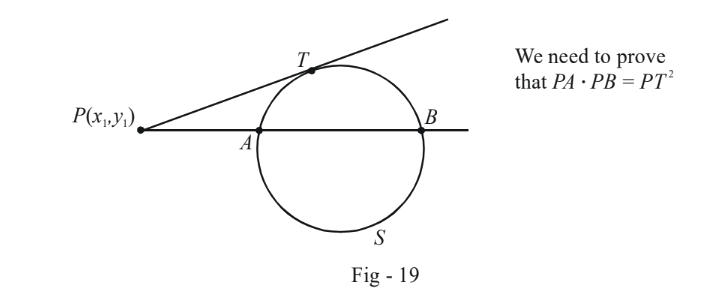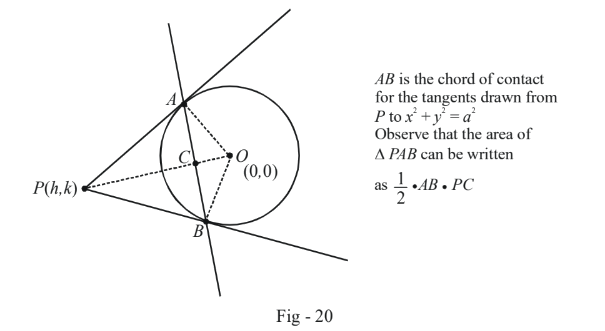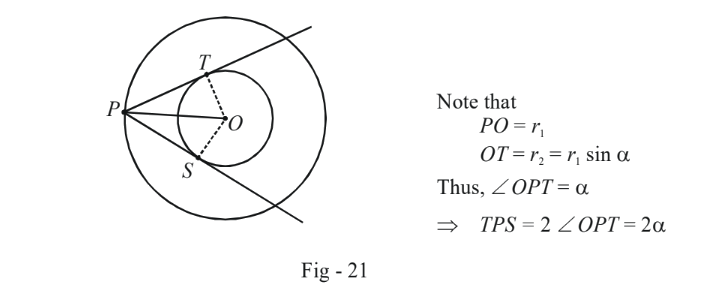# Examples On Tangents To Circles Set-2

Go back to  'Circles'

Example - 20

Refer to Fig - 18. Suppose that $$A$$ and $$B$$, the points of contact of the two tangents, are joined. What will be the equation to this chord of contact ?

Solution: Assume the co-ordinates of $$A$$ and $$B$$ to be $$({x_A},{y_A})$$ and $$({x_B},{y_B})$$ respectively. The equations of the tangents at $$A$$ and $$B$$ are, respectively,

$\left. \begin{array}{l}x{x_A} + y{y_A} + g(x + {x_A}) + f(y + {y_A}) + c = 0\\x{x_B} + y{y_B} + g(x + {x_B}) + f(y + {y_B}) + c = 0\end{array} \right\}\,\,\,\,\,\,\,\,\,\,\,\,\,\,\,\,\,\,\,\,\,\,\,\,\,\,\,\,\,...\left( I \right)$

Since the two tangents intersect at $$P$$, the co-ordinates of $$P$$ must satisfy the system of equations I. Thus,

$\left. \begin{array}{l}{x_1}{x_A} + {y_1}{y_A} + g({x_1} + {x_A}) + f({y_1} + {y_A}) + c = 0\\{x_1}{x_B} + {y_1}{y_B} + g({x_1} + {x_B}) + f({y_1} + {y_B}) + c = 0\end{array} \right\}\,\,\,\,\,\,\,\,\,\,\,\,\,\,\,\,\,\,\,\,\,\,\,\,\,\,\,\,\,\,\,\,\,\,...\left( {II} \right)$

Now, if you observe the system II carefully, you will realise that we can think of it this way: $$({x_A},{y_A})$$ and $$({x_B},{y_B})$$ are two points which satisfy the linear equation

$x{x_1} + y{y_1} + g(x + {x_1}) + f(y + {y_1}) + c = 0 \qquad\qquad\qquad ...(1)$

This is because substitution of $$({x_A},{y_A})$$ or $$({x_B},{y_B})$$ into (1) results in the system of equations II. Thus (1) must be the equation of the chord of contact we are looking for, since both $$A$$ and $$B$$  satisfy (1).

We can write this obtained equation concisely as

$\fbox{\begin{array}{*{20}{c}} {T({x_1},{y_1}) = 0} \end{array}}: \text{ Equation of the chord of contact}$

As an example, the chord of contact for the two tangents drawn from the  origin to the circle

\begin{align}&{x^2} + {y^2} - 4x - 4y + 7 = 0 \;\;will \;\;be\\\\&x{x_1} + y{y_1} + g(x + {x_1}) + f(y + {y_1}) + c = 0\;\; where \;\;({x_1},{y_1}) = (0,0)\\\\\ \Rightarrow \quad & - 2x - 2y + 7 = 0\\\\ \Rightarrow\quad & x + y = \frac{7}{2}\end{align}

Example - 21

From an external point $$P$$, a line is drawn intersecting a circle $$S$$ in two distinct points $$A$$ and $$B$$. A tangent is also drawn from $$P$$ touching the circle $$S$$ at $$T$$. Prove that $$PA \cdot PB$$ is always constant, and equal to $$P{T^2}.$$

Solution: Let the equation of the circle be $$S \equiv {x^2} + {y^2} + 2gx + 2fy + c = 0$$ and the point $$P$$ be $$({x_1},{y_1})$$ :Notice that the line $$PAB$$ passes through the fixed point $$P$$. What is variable about it is its slope, which we assume to be $$\tan \theta$$. Thus, using the polar form for the lines, we obtain the equation of $$PAB$$ as

$\frac{{x - {x_1}}}{{\cos \theta }} = \frac{{y - {y_1}}}{{\sin \theta }} = r\,\,\,\,\,\,\,\,\,\,\,\,\,\,\,\,\,\,\,...(1)$

In particular, the co-ordinates of $$A$$ and $$B$$ can be obtained in terms of $$\theta$$ using the value of $$r$$ as $$PA$$ and $$PB$$ respectively in (1).

Using (1), we can write any point on the line $$PAB$$ as $$({x_1} + r\cos \theta ,\,{y_1} + r\sin \theta ).$$ If this point lies on the circle, it must satisfy the circle’s equation:

\begin{align}{({x_1} + r\cos \theta )^2} + {({y_1} + r\sin \theta )^2} + 2g({x_1} + r\cos \theta ) + 2f({y_1} + r\sin \theta ) + c = 0\\\\ \Rightarrow \qquad {r^2} + (2g\cos \theta + 2f\sin \theta )r + x_1^2 + y_1^2 + 2g{x_1} + 2f{y_1} + c = 0\,\,\,\,\,\,\,\,\,\,\,\,\,\,\,\,\,\,\,\,...(2)\end{align}

The equation (2) in $$r$$ will have two roots $${r_1}$$ and $${r_2}$$ corresponding to $$PA$$ and $$PB$$ since $$A$$ and $$B$$ lie on the circle.

Thus,

\begin{align}{}PA \cdot PB =&\; {r_1} \cdot {r_2} =\; x_1^2 + y_1^2 + 2g{x_1} + 2f{y_1} + c\\\\\,\,\,\,\,\,\,\,\,\,\,\,\,\,\,\,\,\,\,\,\,\,\,\,\,\,\,\,\,\,\,\, =&\; S({x_1},{y_1})\\\\=&\; P{T^2}\ \qquad \qquad \text{(using the result obtained in Example - 17)}\end{align}

You are urged to prove this result using ‘pure’ geometry.

Example - 22

From an external fixed point $$P(h,k),$$ tangents are drawn to the circle $${x^2} + {y^2} = {a^2}$$ . Find the area of the triangle formed by these tangents and their chord of contact.

Solution:Observe that $$\Delta OAC$$ is similar to $$\Delta OAP$$. Thus, the ratio of the corresponding sides is equal.

$\frac{{OC}}{{OA}} = \frac{{AC}}{{AP}} = \frac{{OA}}{{OP}}$

$$OA$$ is simply the radius a, while $$OP$$ is $$\sqrt {{h^2} + {k^2}} .$$ Thus,

$OC = \frac{{O{A^2}}}{{OP}} = \frac{{{a^2}}}{{\sqrt {{h^2} + {k^2}} }}$

Now, $$AC$$ can be evaluated using Pythagoras theorem in $$\Delta OAC:$$

\begin{align}{}AC =&\; \sqrt {O{A^2} - O{C^2}} \\\\\,\,\,\,\,\,\,\,\, =&\; \sqrt {{a^2} - \frac{{{a^4}}}{{{h^2} + {k^2}}}} \\\\\,\,\,\,\,\,\,\,\, =&\; a\sqrt[{}]{{\frac{{{h^2} + {k^2} - {a^2}}}{{{h^2} + {k^2}}}}}\end{align}

The area of $$\Delta PAB\;$$ is

\begin{align}{}\Delta =&\; \frac{1}{2} \times AB \times PC\\\\\,\,\,\,\, =&\; \frac{1}{2} \times (2AC) \times (OP - OC)\\\\\,\,\,\,\, =&\; \frac{{a{{({h^2} + {k^2} - {a^2})}^{3/2}}}}{{{h^2} + {k^2}}}\end{align}

Observe that this question was solved mostly through ‘pure’ geometrical considerations. A pure co-ordinate approach for the area of the triangle can be followed but you can expect it to be much longer. Thus, in this subject, you have to put your intuitive skills to the best use possible to determine the shortest approach.

Let us consider another example here itself which will show why a pure geometrical approach is better sometimes than using co-ordinates.

Consider two circles with the following equations:

$\begin{array}{l}{S_1} \equiv {x^2} + {y^2} + 2gx + 2fy + c = 0\\\\{S_2} \equiv {x^2} + {y^2} + 2gx + 2fy + c{\sin ^2}\alpha + ({g^2} + {f^2}){\cos ^2}\alpha = 0\end{array}$

These two circles are obviously concentric, centred at $$( - g, - f).$$ The radius of $${S_1}$$ is $${r_1} = \sqrt {{g^2} + {f^2} - c}$$ while that of is $${r_2} = \sqrt {{g^2} + {f^2} - c} \sin \alpha = {r_1}\sin \alpha$$ so that $${r_1} > {r_2}.$$

The problem is as follows: from any point on $${S_1},$$ two tangents are drawn to $${S_2}.$$ What is the angle between these two tangents.

Obviously, this angle should be the same for any point chosen on $${S_1}$$ since the circles are perfectly symmetrical figures. Let us draw a diagram corresponding to this situation:As explained in the diagram, the angle between the two tangents can be evaluated by simple geometric considerations to be $$2\alpha .$$ Although this example is more or less trivial, the contrast between pure geometric and co-ordinate approach will become more apparent in some subsequent examples.